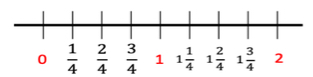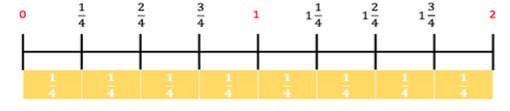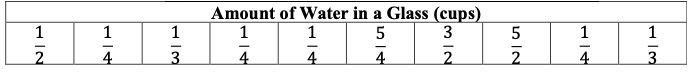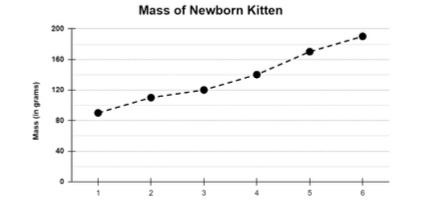# MA.5.DP.1.1Export Print
Collect and represent numerical data, including fractional and decimal values, using tables, line graphs or line plots.

### Examples

Gloria is keeping track of her money every week. She starts with \$10.00, after one week she has \$7.50, after two weeks she has \$12.00 and after three weeks she has \$6.25. Represent the amount of money she has using a line graph.

### Clarifications

Clarification 1: Within this benchmark, the expectation is for an estimation of fractional and decimal heights on line graphs.

Clarification 2: Decimal values are limited to hundredths. Denominators are limited to 1, 2, 3 and 4. Fractions can be greater than one.

General Information
Subject Area: Mathematics (B.E.S.T.)
Strand: Data Analysis and Probability
Status: State Board Approved

## Benchmark Instructional Guide

• Line Graphs
• Line Plots

### Vertical Alignment

Previous Benchmarks

Next Benchmarks

### Purpose and Instructional Strategies

The purpose of this benchmark is to collect and display authentic numerical data in tables, line graphs or line plots, including fractional and decimal values. Students have represented whole number and fractional values using tables, stem-and-leaf plots and line plots in grade 4 (MA.4.DP.1.1). In grade 6, this work will extend to box plots and histograms (MA.6.DP.1.5).
• Instruction with line graphs should develop the understanding that values in this graph often represent data that changes over time.
• Instruction should include identifying the meaning of the points presented on the x-axis and y-axis with both axes being labeled correctly.

### Common Misconceptions or Errors

• For line plots, students may misread a number line and have difficulty because they use whole-number names when counting fractional parts on a number line instead of the fraction name.

### Strategies to Support Tiered Instruction

• Instruction includes opportunities to use concrete models and draw number lines to connect learning with fraction understanding. Students plot fourths on the number line, paying particular attention to what each tick mark and the “distance” between each tick mark represents.
• Example:• For example, utilizing fraction strips or tiles, students will be able to connect fractional parts to the measurement on a number line.Claire studied the amount of water in different glasses. The data she collected is below. Use her data to create a line plot to show the amount of water in the glasses.### Instructional Items

Instructional Item 1

A line graph is shown.• Part A. What is the approximate change in the kitten’s mass, in grams, between Days 3 and 4?
• Part B. What is the approximate change in the kitten’s mass, in grams, between Days 2 and 5?

*The strategies, tasks and items included in the B1G-M are examples and should not be considered comprehensive.

## Related Courses

This benchmark is part of these courses.
5012070: Grade Five Mathematics (Specifically in versions: 2014 - 2015, 2015 - 2022, 2022 and beyond (current))
7712060: Access Mathematics Grade 5 (Specifically in versions: 2014 - 2015, 2015 - 2018, 2018 - 2022, 2022 and beyond (current))
5012065: Grade 4 Accelerated Mathematics (Specifically in versions: 2019 - 2022, 2022 and beyond (current))
5012015: Foundational Skills in Mathematics 3-5 (Specifically in versions: 2019 - 2022, 2022 and beyond (current))

## Related Access Points

Alternate version of this benchmark for students with significant cognitive disabilities.
MA.5.DP.1.AP.1: Sort and represent numerical data, including fractional values using tables or line plots (when given a scaled number line). Data set to include only whole numbers, halves and quarters.

## Related Resources

Vetted resources educators can use to teach the concepts and skills in this benchmark.

## Formative Assessment

Chores Data:

Students are asked to display numerical data on a dot plot.

Type: Formative Assessment

## Lesson Plans

Voter Turnout and the 26th Amendment:

In this lesson plan, students will graph and interpret voter turnout data to explain how the 26th Amendment expanded the opportunity for civic participation.

Type: Lesson Plan

Voter Turnout and the 24th Amendment:

Students will use voter turnout data to create and analyze a line graph to explain how the 24th Amendment expanded civic participation in this integrated lesson plan.

Type: Lesson Plan

Voter Turnout and the 19th Amendment:

Students will graph and analyze voting data to explain how the 19th Amendment expanded civic participation in this lesson plan.

Type: Lesson Plan

Voter Turnout and the 15th Amendment:

In this lesson plan, students will graph and analyze voting data to explain how the 15th Amendment expanded civic participation.

Type: Lesson Plan

Heatin' It Up or Coolin' It Down:

Many chemical reactions are accompanied by a change in temperature. Whether it is extreme or barely noticeable, the temperature may go up or it may go down. Investigate these two chemical reactions described in this lesson to experience two different kinds of temperature change.

Type: Lesson Plan

Line Plotting with Fractions Chicago Pizza Style:

In this lesson, students will be making a line plot and recording fractional data (1/4, 1/2, and 3/4) on their line plot. Students will then use fraction operations to solve problems involving data presented in their line plots.

Type: Lesson Plan

April Showers Bring May Flowers - Line Plots:

In this lesson, students will create a line plot that displays rainfall data.  They will use the data from the line plot to answer questions using addition, subtraction, multiplication, and division of fractions. Please note that the denominators for the line plot go to eighths.

Type: Lesson Plan

What is in the PLOT?:

In this lesson students will use Florida's wonderful seashells to collect data sets of measurement in fractions of a unit (1/2, 1/4, 1/8). They will plot the data on a line plot, then use operations with fractions to solve problems involving information presented in the line plot.

Type: Lesson Plan

Sleep Versus Mood:

Using the case study, No Sleep, No Problem? Students will explore the amount of sleep they are allotting for themselves per night. Students will then investigate the impact of sleep on one’s daily mood.

Type: Lesson Plan

## Original Student Tutorials

Grasshopper Hut for Gus: Line Plots:

Help build a Grasshopper Hut for Gus by creating line plots and answering questions about the line plots in this interactive tutorial.

Type: Original Student Tutorial

Learn to interpret data presented on a line plot and use operations on fractions to solve problems involving information presented in line plots as you complete this beach-themed, interactive tutorial.

Type: Original Student Tutorial

## MFAS Formative Assessments

Chores Data:

Students are asked to display numerical data on a dot plot.

## Original Student Tutorials Mathematics - Grades K-5

Grasshopper Hut for Gus: Line Plots:

Help build a Grasshopper Hut for Gus by creating line plots and answering questions about the line plots in this interactive tutorial.

Learn to interpret data presented on a line plot and use operations on fractions to solve problems involving information presented in line plots as you complete this beach-themed, interactive tutorial.

## Student Resources

Vetted resources students can use to learn the concepts and skills in this benchmark.

## Original Student Tutorials

Grasshopper Hut for Gus: Line Plots:

Help build a Grasshopper Hut for Gus by creating line plots and answering questions about the line plots in this interactive tutorial.

Type: Original Student Tutorial